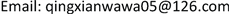1重庆市计量质量检测研究院，重庆

2重庆市公安局，重庆1. 引言

2. 国内外研究情况

3. 工作原理

4. 装置组成

4.1. 隔离与信号调理电路

4.2. 电压调理电路

U p e a k = 0.25 × R 6 + R 7 + R 8 + R 9 R 9 = 381.6   V (1)

4.3. 电流调理电路

U p e a k = 0.25 R 1 = 5   A (2)

k v = R 9 R 6 + R 7 + R 8 + R 9 × 8.2 × R 12 R 10 = 0.02435 (3)

k i = R 1 × 8.2 × R 12 R 10 = 1.8587 (4)

5. 仿真5.1. 仿真电路图

5.2. 仿真波形

5.3. 放电过程能量计算

5.3.1. 理论计算值

E 理 论 = 0.5 C 1 U 2 + 0.5 C 2 U 2 = 0.5 × 0.47 × 10 − 6 × 310.9998 2   J + 0.5 × 4.7 × 10 − 6 × 310.9998 2   J = 250.0232   mJ

5.3.2. 矩形法计算

E 计 算 = Δ t R × [ ∑ i = 1 n U i 2 ] = 6.066 × 10 − 6 2000 × ( 310.9998 2 + 310.8474 2 + 310.7041 2 + ⋯ ) = 250.6792   mJ

5.3.3. 梯形法计算

E 计 算 = Δ t 2 R × [ U 1 2 + 2 ∑ i = 2 n U i 2 ] = 6.066 × 10 − 6 2 × 2000 × [ 310.9998 2 + 2 × ( 310.8474 2 + 310.7041 2 + 310.5560 2 + ⋯ ) ] = 250.5254   mJ

5.3.4. 辛普森法计算

E 计 算 = Δ t 3 R × [ U 1 2 + 4 ∑ i = 1 n U 2 i 2 + 2 ∑ i = 1 n U 2 i + 1 2 ] = 6.066 × 10 − 6 3 × 2000 × [ 310.9998 2 + 4 × ( 310.8474 2 + 310.5560 2 + ⋯ )     + 2 × ( 310.7041 2 + 310.4084 2 + ⋯ ) ] = 250.5253   mJ

5.3.5. 科特斯法计算

E 计 算 = 2 Δ t 45 R × [ 7 U 1 2 + 32 ∑ i = 0 n U 4 i + 2 2 + 12 ∑ i = 0 n U 4 i + 3 2 + 32 ∑ i = 0 n U 4 i + 4 2 + 14 ∑ i = 0 n U 4 i + 5 2 ] = 2 × 6.066 × 10 − 6 45 × 2000 × [ 7 × 310.9998 2 + 32 × ( 310.8474 2 + 310.2607 2 + ⋯ )     + 12 × ( 310.7041 2 + 310.1131 2 + ⋯ ) + 32 × ( 310.5560 2 + 309.9645 2 + ⋯ )     + 14 × ( 310.4084 2 + 309.8182 2 + ⋯ ) ] = 250.5253   mJ

5.3.6. 误差计算

6. 结论